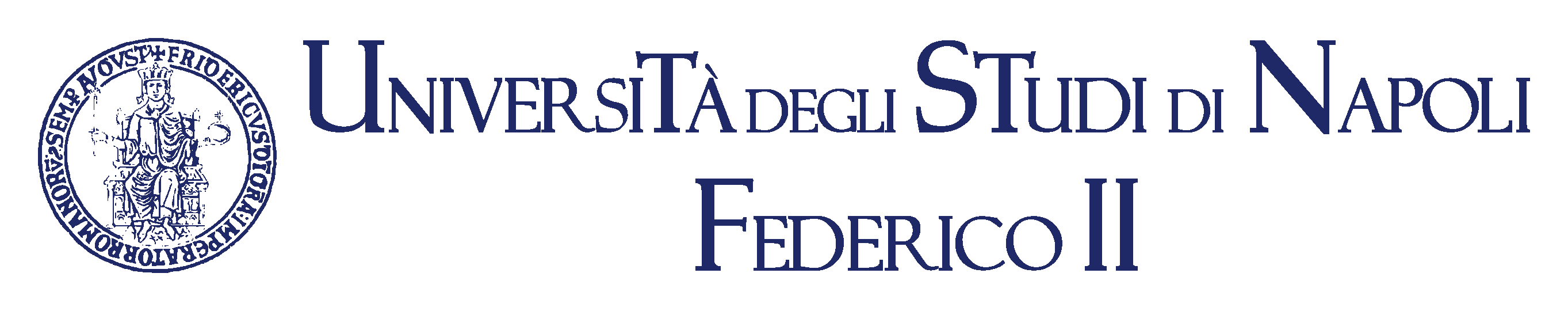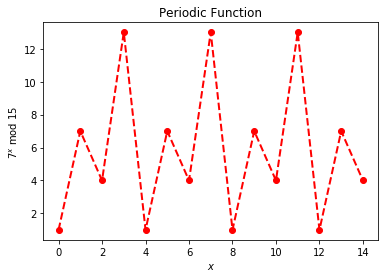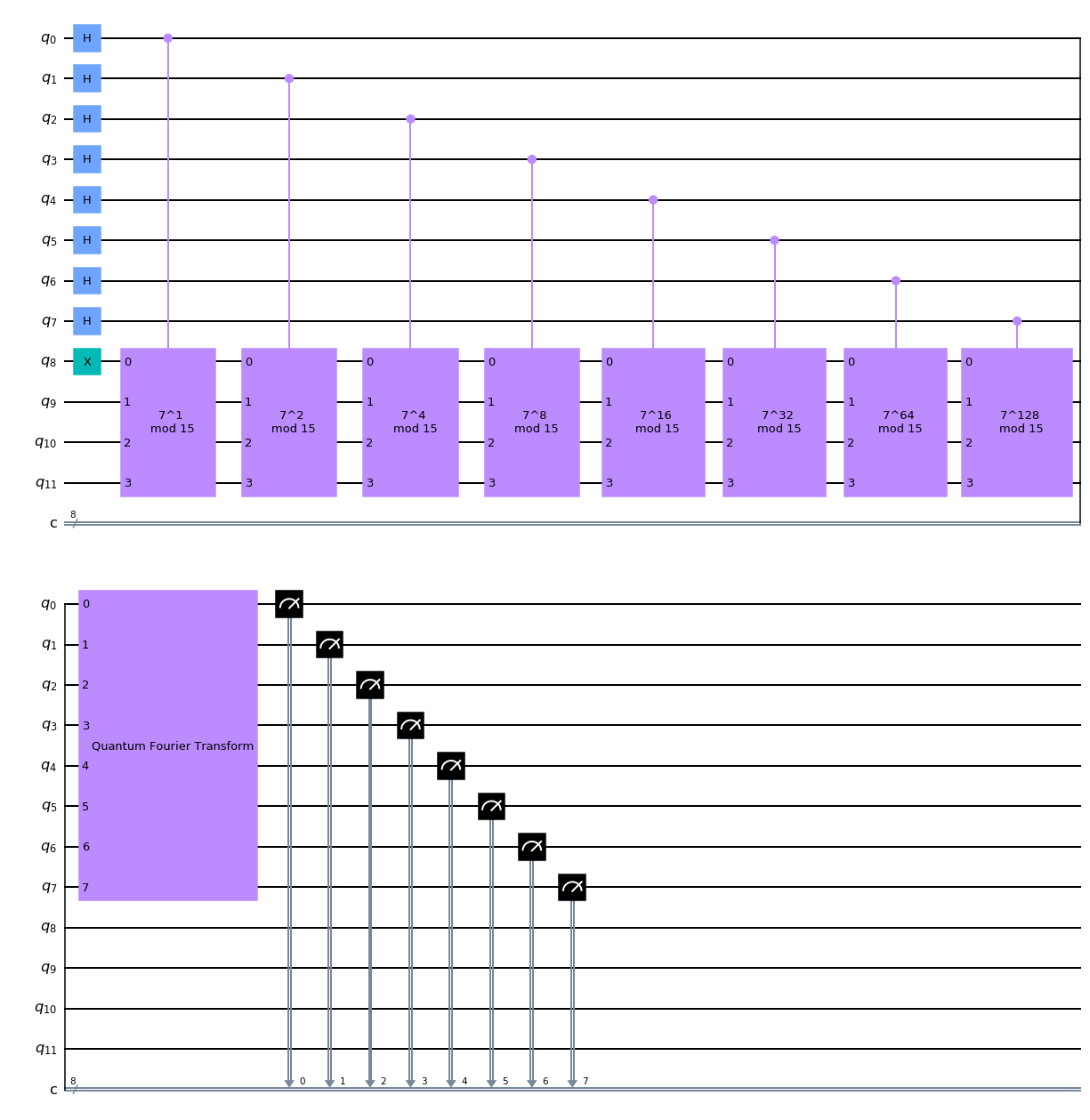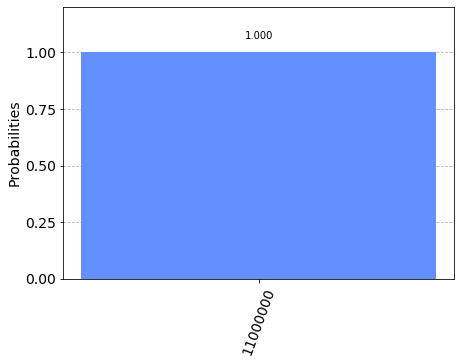In :
from IPython.display import Image
from IPython.core.display import display, HTML
Image("images/F2.png",width=300)

Out:In :
Image("images/dieti.png",width=200)

Out:# Introduction to Quantum Circuits¶

## Shor's Algorithm¶

In :
import matplotlib.pyplot as plt
import numpy as np
from qiskit import QuantumCircuit, Aer, execute
from qiskit.visualization import plot_histogram, plot_bloch_multivector, plot_state_city
from math import gcd
from numpy.random import randint
import pandas as pd
from fractions import Fraction
print("Imports Successful")

Imports Successful


## Period Finding¶

Let’s consider the periodic function of $x$:

$$f(x) = 7^x \bmod{15}$$
In :
N = 15
a = 7

# Calculate the plotting data
xvals = np.arange(15)
yvals = [np.mod(a**x, N) for x in xvals]

fig, ax = plt.subplots()
ax.plot(xvals, yvals, linewidth=2, color='red', linestyle='dashed', marker='o')
ax.set(xlabel='$x$', ylabel='$%i^x$ mod $%i$' % (a, N),title="Periodic Function")

Out:
[Text(0, 0.5, '$7^x$ mod $15$'),
Text(0.5, 0, '$x$'),
Text(0.5, 1.0, 'Periodic Function')]## Shor Algorithm Circuit¶

The operator $U$ is defined as:

$$U|y\rangle = |7 \times y\bmod 15\rangle$$

To create $U^x$, we will simply repeat the circuit $x$ times

$$U^x|y\rangle = |7^x \times y\bmod 15\rangle = U U U \ldots U|y\rangle$$

Please refer to the

In :
def c7xmod15(power):

U = QuantumCircuit(4)
#repeat the definition of U x times using a "for" loop
for iteration in range(power):
"""Controlled multiplication by 7 mod 15"""
U.x(0)
U.x(1)
U.x(2)
U.x(3)
U.cx(1,2)
U.cx(2,1)
U.cx(1,2)
U.cx(2,3)
U.cx(3,2)
U.cx(2,3)
U.cx(0,3)
U.cx(3,0)
U.cx(0,3)
U = U.to_gate()
U.name = "%i^%i \n mod 15" % (7, power)
c_U = U.control()
return c_U

In :
# Specify variables
n_count = 8 # number of counting qubits
a = 7


Circuit for the Quantum Fourier Transform

In :
def qft(n):
"""n-qubit QFT"""
qc = QuantumCircuit(n)
for j in range(n):
qc.h(j)
for m in range(j):
qc.cp(np.pi/float(2**(j-m)), m, j)
for qubit in range(n//2):
qc.swap(qubit, n-qubit-1)
# the Swap gates perform the permutation P of the bits required by the definition of QFT.
qc.name = "Quantum Fourier Transform"

return qc

In :
# Create QuantumCircuit that perform Shor algorithm
# with n_count counting qubits |x> plus 4 qubits |y> for U

qc = QuantumCircuit(n_count + 4, n_count)

##################################
# INIZIALIZE |x> and |y> registers
##################################

# Apply Hadamard to all |x> qubits
for q in range(n_count):
qc.h(q)

# The quantum register |y> is inizializated to the state y = |0001>
qc.x(n_count)

##################################
# Do controlled-U^(2^q) operations
##################################
for q in range(n_count):
qc.append(c7xmod15(2**q),
[q] + [i+n_count for i in range(4)])

##################################
# Quantum Fourier Transform
##################################

qc.append(qft(n_count), range(n_count))

# Measurement on the counting qubits
qc.measure(range(n_count), range(n_count))

qc.draw('mpl')

Out:In :
backend = Aer.get_backend('qasm_simulator')

# Do a 1 shot simulation
results = execute(qc, backend, shots=1).result()
# returns the counts
counts = results.get_counts()
plot_histogram(counts)

Out:Since we have 3 qubits, these results correspond to measured phases of:

In :
rows, measured_phases = [], []
for output in counts:
decimal = int(output, 2)  # Convert (base 2) string to decimal
phase = decimal/(2**n_count)
# In Mermin's notation this quantity is h/r where h is an integer and r is the period
measured_phases.append(phase)
# Add these values to the rows in our table:
rows.append(["%s(bin) = %i(dec)" % (output, decimal),
"%i/%i = %.2f" % (decimal, 2**n_count, phase)])
# Print the rows in a table
headers=["Register Output", "Phase"]
df = pd.DataFrame(rows, columns=headers)
print(df)

            Register Output           Phase
0  11000000(bin) = 192(dec)  192/256 = 0.75

In :
rows = []
for phase in measured_phases:
frac = Fraction(phase).limit_denominator(15)
# The function "Fraction" returns the two integer whose ratio is phase = h/r
rows.append([phase, "%i/%i" % (frac.numerator, frac.denominator), frac.denominator])
# Print as a table
headers=["Phase", "Fraction", "Guess for r"]
df = pd.DataFrame(rows, columns=headers)
print(df)

   Phase Fraction  Guess for r
0   0.75      3/4            4

1. This jupyter notebook has been adapted from https://qiskit.org/textbook/ch-algorithms/shor.html

2. P.W. Shor, Polynomial-time algorithms for prime factorization and discrete logarithms on a quantum computer, SIAM review, 1999 - SIAM

3. M. Nielsen and I. Chuang, Quantum Computation and Quantum Information, Cambridge Series on Information and the Natural Sciences (Cambridge University Press, Cambridge, 2000).

4. N. David Mermin "Quantum Computer Science (An Introduction)", Cambridge (2007)

5. Qiskit: An Open-source Framework for Quantum Computing (2019), doi:10.5281/zenodo.2562110